# GSEB Solutions Class 8 Maths Chapter 6 Square and Square Roots Ex 6.3

Gujarat Board GSEB Textbook Solutions Class 8 Maths Chapter 6 Square and Square Roots Ex 6.3 Textbook Questions and Answers.

## Gujarat Board Textbook Solutions Class 8 Maths Chapter 6 Square and Square Roots Ex 6.3

Question 1.
What could be the possible ‘ones’ digit of the square root of each of the following numbers?

1. 9801
2. 99856
3. 998001
4. 657666025

Solution:
The possible digit at ones place of the square root of:
1. 9801 can be 1 or 9.
[∵1 × 1 = land 9 × 9 = 81]

2. 99856 can be 4 or 6.
[∵ 4 × 4 = 16 and 6 × 6 = 36]

3. 998001 can be 1 or 9.

4. 657666025 can be 5. [∵ 5 × 5 = 25]Question 2.
Without doing any calculation, find the numbers which are surely not perfect squares?

1. 152
2. 257
3. 408
4. 441

Solution:
We know that the ending digit of perfect square is 0, 1, 4, 5, 6, and 9.
∴ A number ending in 2, 3, 7 or 8 can never be a perfect square.
1. 153, cannot be a perfect square.

2. 257, cannot be a perfect square.

3. 408, cannot be a perfect square.

4. 441, can be a perfect square.
Thus, (i) 153, (ii) 257 and (iii) 408 are surely not perfect squares.

Question 3.
Find the square roots of 100 and 169 by the method of repeated subtraction?
Solution:
1. $$\sqrt{100}$$
We have 100 – 1 = 99
99 – 3 = 96
96 – 5 = 91
91 – 7 = 84
84 – 9 = 75
75 – 11 = 64
64 – 13 = 51
51 – 15 = 36
36 – 17 = 19
19 – 19 = 0
∵ We reach at 0 by successive subtraction of 10 odd numbers.
∴ $$\sqrt{100}$$ = 10

2. $$\sqrt{169}$$
We have
169 – 1 = 168
168 – 3 = 165
165 – 5 = 160
160 – 7 = 153
153 – 9 = 144
144 – 11 = 133
133 – 13 = 120
120 – 15 = 105
105 – 17 = 88
88 – 19 = 69
69 – 21 = 48
48 – 23 = 25
25 – 25 = 0
∵ We reach at 0 by successive subtraction of 13 odd numbers.
∴ $$\sqrt{169}$$ = 13.Question 4.
Find the square root of the following numbers by the Prime Factorisation Method?

1. 729
2. 400
3. 1764
4. 4096
5. 7744
6. 9604
7. 5929
8. 9216
9. 529
10. 8100

Solution:
1. We have 729 = 3 × 3 × 3 × 3 × 3 × 3
∴ $$\sqrt{729}$$ = 3 × 3 × 3 = 27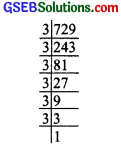Thus, the square root of 729 = 27.

2. We have 400 = 2 × 2 × 2 × 2 × 5 × 5∴ $$\sqrt{400}$$ = 2 × 2 × 5 = 20

3. We have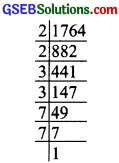1764 = 2 × 2 × 3 × 3 × 7 × 7
∴ $$\sqrt{1764}$$ = 2 × 3 × 7 = 42

4. We have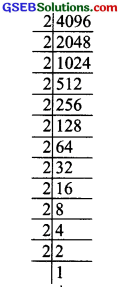4096 = 2 × 2 × 2 × 2 × 2 × 2 × 2 × 2 × 2 × 2 × 2 × 2
∴ $$\sqrt{4096}$$ = 2 × 2 × 2 × 2 × 2 × 2 = 64
Thus, the square root of 4096 is 64.

5. We have
7744 = 2 × 2 × 2 × 2 × 2 × 2 × 11 × 11
∴ $$\sqrt{7744}$$ = 2 × 2 × 2 × 11 = 88
Thus, the square root of $$\sqrt{7744}$$ is 88.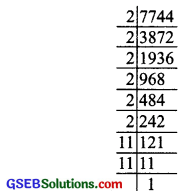6. We have
9604 = 2 × 2 × 7 × 7 × 7 × 7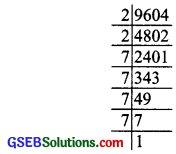∴ $$\sqrt{9604}$$ = 2 × 7 × 7 = 98
Thus, the square root of 9604 is 98.

7. We have
5929 = 7 × 7 × 11 × 11∴ $$\sqrt{5929}$$ = 7 × 11 = 77
Thus, the square root of 5929 is 77.

8. We have
9216 = 2 × 2 × 2 × 2 × 2 × 2 × 2 × 2 × 2 × 2 × 3 × 3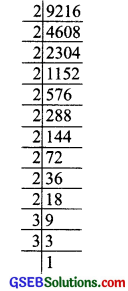∴ $$\sqrt{9216}$$ = 2 × 2 × 2 × 2 × 2 × 3 = 96
Thus, the square root of 9216 is 96.

9. We have
529 = 23 × 23
∴ $$\sqrt{529}$$ = 23Thus, the square root of 529 is 23

10. We have
8100 = 2 × 2 × 3 × 3 × 3 × 3 × 5 × 5
∴ $$\sqrt{8100}$$ = 2 × 3 × 3 × 5 = 90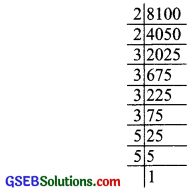Thus, the square root of 8100 is 90.Question 5.
For each of the following numbers, find the smallest whole number by which it should be multiplied so as to get a perfect square number. Also find the square root of the square siumber so obtained?

1. 252
2. 180
3. 1008
4. 2028
5. 1458
6. 768

Solution:
1. We have 252 = 2 × 2 × 3 × 3 × 7
∵ The prime factor 7 has no pair
∴  × 7 = [2 × 2 × 3 × 3 × 7 × 7]
or 1764 = 2 × 2 × 3 × 3 × 7 × 7
and $$\sqrt{1764}$$ = 2 × 3 × 7 = 42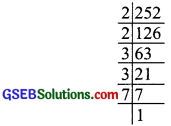Thus, the required smallest whole number = 7
Also, the square roo4 of 1764 is 42.

2. We have 180 = 2 × 2 × 3 × 3 × 5∵ The prune factor 5 has no pair.
∴  × 5 = (2 × 2 × 3 × 3 × 5] × 5
or 900 = 2 × 2 × 3 × 3 × 5 × 5
or $$\sqrt{900}$$ = 2 × 3 × 5 = 30
Thus, the required smallest whole number = 5
Also, the square root of 900 is 30,

3. We have
1008 = 2 × 2 × 2 × 2 × 3 × 3 × 7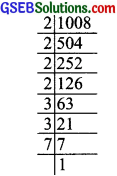∵ The prime factor 7 has no pair.
∴ (1008) × 7 = [2 × 2 × 2 × 2 × 3 × 3 × 7] × 7
or 7056 = 2 × 2 × 2 × 2 × 3 × 3 × 7 × 7
or $$\sqrt{7056}$$ = 2 × 2 × 3 × 7 = 84
Thus, the required smallest whole number = 7
Also the square root of 7056 is 84.

4. We have
2028 = 2 × 2 × 3 × 3 × 3
∵ The prime factor 3 has no pair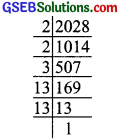∴  × 3 = [2 × 2 × 3 × 13 × 13] × 3
0r 6084 = 2 × 2 × 3 × 3 × 13 × 13
or $$\sqrt{6084}$$ = 2 × 3 × 13 = 78
Thus, the smallest whole number = 3 and $$\sqrt{6084}$$ = 78

5. We have
1458 = 2 × 3 × 3 × 3 × 3 × 3 × 3
∵ 2 has no pair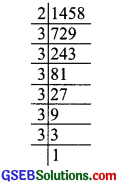∴  × 2 = [2 × 3 × 3 × 3 × 3 × 3 × 3] × 2
or 2916 = 2 × 2 × 3 × 3 × 3 × 3 × 3 × 3
or $$\sqrt{2916}$$ = 2 × 3 × 3 × 3 = 54
Thus, the required smallest whole number = 2, and $$\sqrt{2916}$$ = 54

6. We have
768 = 2 × 2 × 2 × 2 × 2 × 2 × 2 × 2 × 3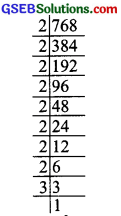∵ Prime factor 3 has no pair.
∴  × 3 = [2 × 2 × 2 × 2 × 2 × 2 × 2 × 2 × 3] × 3
2304 = 2 × 2 × 2 × 2 × 2 × 2 × 2 × 2 × 3 × 3
or $$\sqrt{2304}$$ = 2 × 2 × 2 × 2 × 3 = 48
Thus, the smallest whole number = 3 and
$$\sqrt{2304}$$ = 48Question 6.
For each of the following numbers, find the smallest whole number by which it should be divided so as to get a perfect square. Also find the square mot of the square number so obtained?

1. 252
2. 2925
3. 396
4. 2645
5. 21100
6. 1620

Solution:
1. 252
We have 252 = 2 × 2 × 3 × 3 × 7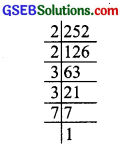∵ The prime factor 7 is unpaired, so the given number should be divided by 7.
∴ $$\frac{252}{7}$$ = $$\frac{2×2×3×3×7}{7}$$
or 36 = 2 × 2 × 3 × 3
Thus, 36 is a perfect square and $$\sqrt{36}$$ = 2 × 3 = 6

2. 2925
We have
2925 = 3 × 3 × 3 × 5 × 13∵ The prime factor 13 is unpaired, so the given number should be divided by 13.
∴ $$\frac{2925}{13}$$ = $$\frac{3×3×5×5×13}{13}$$
or 225 = 3 × 3 × 5 × 5
Thus, 225 is a perfect square and $$\sqrt{225}$$ = 3 × 5 = 15

3. 396
We have
396 = 2 × 2 × 3 × 3 × 11
As the prime factor 11 is unpaired.∴ Dividing the given number by 11,
$$\frac{396}{11}$$ = $$\frac{2×2×3×3×11}{11}$$
36 = 2 × 2 × 3 × 3
Thus, 36 is a perfect square and $$\sqrt{36}$$ = 2 × 3 = 6

4. 2645
We have
2645 = 5 × 23 × 23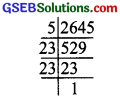∵ The prime factor 5 is unpaired.
∴ Dividing the given number by 5, we have
∴ $$\frac{2645}{5}$$ = $$\frac{5×23×23}{5}$$
or 529 = 23 × 23
Thus, 529 is a perfect square, and $$\sqrt{529}$$ = 23.

5. 2800
We have
2800 = 2 × 2 × 2 × 2 × 5 × 5 × 7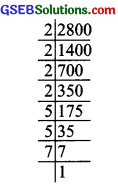Since, 7 is unpaired.
∴ The given number should be divided by 7.
i.e; $$\frac{2800}{7}$$ = $$\frac{2 \times 2 \times 2 \times 2 \times 5 \times 5 \times 7}{7}$$
or 400 = 2 × 2 × 2 × 2 × 5 × 5
which is a perfect square
∴ $$\sqrt{400}$$ = 2 × 2 × 5 = 20

6. 1620
We have
1620 = 2 × 2 × 3 × 3 × 3 × 3 × 5
∵ The pnme factor 5 is unpaired.
∴ Dividing the given number by 5,
we have $$\frac{1620}{5}$$ = $$\frac{2 \times 2 \times 3 \times 3 \times 3 \times 3 \times 5}{5}$$
0r 324 = 2 × 2 × 3 × 3 × 3 × 3
Thus, $$\sqrt{324}$$ = 2 × 3 × 3 = 18Question 7.
The students of Class VIII of a school donated ₹2401 in all, for Prime Minister’s National Relief Fund Each student donated as many rupees as the number of students in the class. Find the number of students in the class?
Solution:
Let the number of students = x
∴ Each student donated ₹x.Total amount donated by the 7343
class = ₹x × x = ₹x2
Thus, x2 = 2401
or $$\sqrt{x^{2}}$$ = $$\sqrt{2401}$$
or x = $$\sqrt{2401}$$ = $$\sqrt{7×7×7×7}$$ = 7 × 7 = 49
∴ The number of students in the class 49.

Question 8.
2025 plants we so be planted ¡n a garden in such a way that each row contains as many plants as the number of rows. Find the number of rows and the number of plants in each row?
Solution:
Let the number of rows = x
∴ Number of plants is a row = x
So, the number of plants to be planted
= x × x = x2
∴ x2 = 2025 or $$\sqrt{x^{2}}$$ = $$\sqrt{2025}$$
or x = $$\sqrt{3 × 3 × 3 × 3 × 5 × 5}$$
= 3 × 3 × 5 = 45Thus, the required number of rows = 45
Also, number of plants in a row = 45

Question 9.
Find the smalkst square number that is divisible by each of the numbers 4, 9 and 10.
Solution:
We know that LCM is the smallest number divisible by all its factors.
Since, LCM of 4, 9 and 10 = 2 × 2 × 9 × 5
= 180But 180 is not a perfect square
Again,
180 = 2 × 2 × 3 × 3 × 5
[∵9 = 3 × 3]
∵ It has 5 as unpaired.
∴  × 5 = [2 × 2 × 3 × 3 × 5] × 5 or 900 = 2 × 2 × 3 × 3 × 5 × 5
∵All die prime factors of 900 are paired.
∴ 900 is a perfect square.
Thus, the required number 900.Question 10.
Find the satallest square number that is divisible by each of the numbers 8, 15 and 20?
Solution:
The smallest number divisible by 8, 15 and 20 is their L.CM.
We have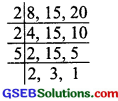LCM = 2 × 2 × 5 × 2 × 3
= 120
But 120 is not a square number.
Now, to make it ii perfect square. we have
120 = 2 × 2 × 2 × 3 × 5
or  × 2 × 3 × 5
= [2 × 2 × 2 × 3 × 5] × 2 × 3 × 5
or 3600 = 2 × 2 × 2 × 2 × 3 × 3 × 5 × 5
All factors of 3600 are paired. Therefore, 3600 is a perfect square.
Thus, the required number = 3600.